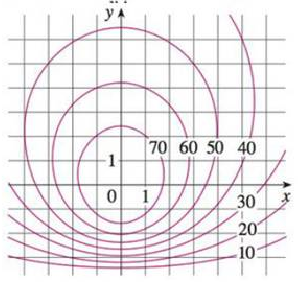Chapter 14.1, Problem 33E

Chapter
Section
Textbook Problem

A contour map for a function f is shown. Use it to estimate the values of f(−3, 3) and f(3, −2). What can you say about the shape of the graph?To determine

To estimate: The values of f(3,3) and f(3,2) from the given contour map of the function f.

Explanation

It is observed from the given contour map that the point (−3, 3) lies between the level curves of z values 50 and 60.

Thus, it can be concluded that the value of f(3,3)56

Still sussing out bartleby?

Check out a sample textbook solution.

See a sample solution

The Solution to Your Study Problems

Bartleby provides explanations to thousands of textbook problems written by our experts, many with advanced degrees!

Get Started

Find f g h. 39. f(x) = 3x 2, g (x) = sin x, h(x) = x2

Single Variable Calculus: Early Transcendentals, Volume I

Sketch the graphs of the equations in Exercises 512. 2yx2=1

Finite Mathematics and Applied Calculus (MindTap Course List)

Simplify: a. 64x639y2x6;x0 b. (a3b4)2(ba)3

Applied Calculus for the Managerial, Life, and Social Sciences: A Brief Approach

In problems 23-58, perform the indicated operations and simplify. (x3+x1)(x+2)

Mathematical Applications for the Management, Life, and Social Sciences

Subtract: (0)(4)

Elementary Technical Mathematics

For y = 3x, y = a) 3x log3 e b) 3x ln 3 c) 3xlog3e d) 3xln3

Study Guide for Stewart's Single Variable Calculus: Early Transcendentals, 8th

For , f′(x) =

Study Guide for Stewart's Multivariable Calculus, 8th